# Group cohomology

<]]>

<]]>
• ## Online References

nlab, a bit nonclassical

<]]>
• ## Paper References

Godement, Sem Bourbaki exp 90: Cohomology of discontinuous groups

Various things in the folder Group and Galois cohomology, including book by Brown and historical survey by MacLane.

Cam Studies in Adv Math 31: Representations and cohomology II, by Benson, excellent. What about vol I??

<]]>
• ## Definition

One can define group cohomology as a sheaf cohomology. For this, one considers the site given by the canonical topology on the category of left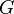-sets. Abelian sheaves on this site are equivalent to left-modules. For a left G-module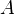, the cohomology group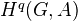can be expressed as the sheaf cohomology group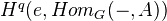, where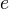is the one-point set with its unique structure of a left-set. There is also a more general expression for a subgroup of.

For continuous group cohomology of a profinite group (Tate cohomology?), one has a similar story. See Tamme p. 55-56, for details.

One can view group cohomology as a functor from modules with a group action to abelian groups. See Baues: Homotopy types, section 5.

There is also a notion of group hypercohomology ("coeffs in a complex of modules").

<]]>

<]]>

<]]>

<]]>

<]]>

<]]>

<]]>

<]]>

<]]>